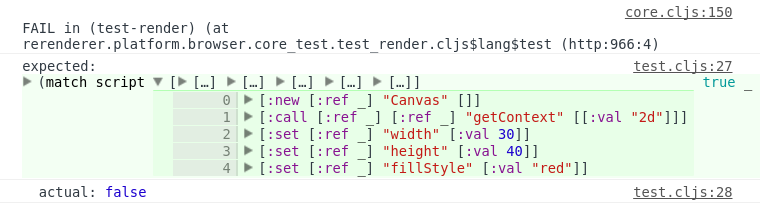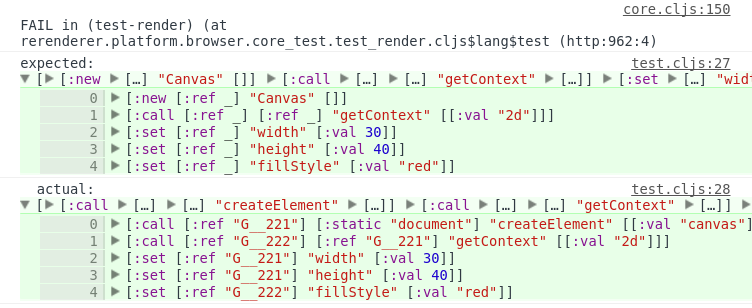Sometimes I need to test content of a big structure, where not all content meaningful, so I need something like fuzzy assertions. And I use core.match for that, like:

``````(ns example.test
(:require [cljs.test :refer-macros [deftest is]]
[cljs.core.match :refer-macros [match]]
[example.core :refer [get-script]]))

(deftest test-render
(let [script (get-script)]
(is (match script
[[:new [:ref _] "Canvas" []]
[:call [:ref _] [:ref _] "getContext" [[:val "2d"]]]
[:set [:ref _] "width" [:val 30]]
[:set [:ref _] "height" [:val 40]]
[:set [:ref _] "fillStyle" [:val "red"]]] true
_ false))))
``````

But it’s redundant, and failure messages are a bit ugly and it doesn’t even show content of not matched structure:That can be easily fixed, because `cljs.test` is very extendable. So we just need to create macros for simpler matching and implement `assert-expr` method for it:

``````(ns example.test-utils
(:require [cljs.core.match :refer [match]]
[cljs.test :refer [assert-expr]]))

(defmacro match?
[x pattern]
`(match ~x
~pattern true
_# false))

(defmethod assert-expr 'match? [_ msg form]
(let [[_ x pattern] form]
`(if ~form
(cljs.test/do-report {:type :pass
:message ~msg
:expected '~form
:actual nil})
(cljs.test/do-report {:type :fail
:message ~msg
:expected '~pattern
:actual ~x}))))
``````

Then update test:

``````(ns example.test
(:require [cljs.test :refer-macros [deftest is]]
[example.test :refer-macros [match?]]
[example.core :refer [get-script]]))

(deftest test-render
(let [script (get-script)]
(is (match? script
[[:new [:ref _] "Canvas" []]
[:call [:ref _] [:ref _] "getContext" [[:val "2d"]]]
[:set [:ref _] "width" [:val 30]]
[:set [:ref _] "height" [:val 40]]
[:set [:ref _] "fillStyle" [:val "red"]]]))))
``````

After that failure message will be much nicer: